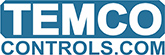# Bit wise AND/OR operation

Can we store binary data in variables ?
And can we do bit wise AND/OR operations on this ?

Yes of course, you can set the range to any of the binary ranges like on/off or open/closed.

Then you can do your usual logic on these variables.

IF FAN OR PUMP THEN SYSTEM = ON

IF NOT FAN AND NOT PUMP THEM SYSTEM = OFF

A bad example showing you can use AND and OR in your logic.

1 Like

Thanks Maurice,
What I want to ask is that can I change bits in registers, e.g. if I want to change 8th bit of 32 bit register from 0 to 1

Sorry, we don’t have any bitwise functions but with a bit of work you could use integer math to get many of the functions. I found this discussion about the topic, not all operations are possible and the methods use some tricks we don’t have like for-next-loops. If it’s a big feature for you maybe we can get it into the todo list.

https://stackoverflow.com/questions/2982729/is-it-possible-to-implement-bitwise-operators-using-integer-arithmetic1 Like

Sure, I understand you would like to do some bitwise functions. We can add it to the todo list… which is pretty long already but its in there.1 Like

Thanks Maurice, I have developed a script to manipulate last 8 bits.

10 ALMTEST = ALMTEST2
20 IF BITCHAN8 = 1 AND ALMTEST < 255 THEN 8TH_BIT = 1 , ALMTEST2 = ALMTEST2 + 128
30 IF BITCHAN8 = 0 AND ALMTEST >= 255 THEN 8TH_BIT = 0 , ALMTEST2 = ALMTEST2 - 128
40 IF ALMTEST - 128 > 0 THEN ALMTEST = ALMTEST - 128
50 IF BITCHAN7 = 1 AND ALMTEST < 127 THEN 7TH_BIT = 1 , ALMTEST2 = ALMTEST2 + 64
60 IF BITCHAN7 = 0 AND ALMTEST >= 127 THEN 7TH_BIT = 0 , ALMTEST2 = ALMTEST2 - 64
70 IF ALMTEST - 64 > 0 THEN ALMTEST = ALMTEST - 64
80 IF BITCHAN6 = 1 AND ALMTEST < 63 THEN 6TH_BIT = 1 , ALMTEST2 = ALMTEST2 + 32
90 IF BITCHAN6 = 0 AND ALMTEST >= 63 THEN 6TH_BIT = 0 , ALMTEST2 = ALMTEST2 - 32
100 IF ALMTEST - 32 > 0 THEN ALMTEST = ALMTEST - 32
110 IF BITCHAN5 = 1 AND ALMTEST < 31 THEN 5TH_BIT = 1 , ALMTEST2 = ALMTEST2 + 16
120 IF BITCHAN5 = 0 AND ALMTEST >= 31 THEN 5TH_BIT = 0 , ALMTEST2 = ALMTEST2 - 16
130 IF ALMTEST - 16 > 0 THEN ALMTEST = ALMTEST - 16
140 IF BITCHAN4 = 1 AND ALMTEST < 15 THEN 4TH_BIT = 1 , ALMTEST2 = ALMTEST2 + 8
150 IF BITCHAN4 = 0 AND ALMTEST >= 15 THEN 4TH_BIT = 0 , ALMTEST2 = ALMTEST2 - 8
160 IF ALMTEST - 8 > 0 THEN ALMTEST = ALMTEST - 8
170 IF BITCHAN3 = 1 AND ALMTEST < 7 THEN 3RD_BIT = 1 , ALMTEST2 = ALMTEST2 + 4
180 IF BITCHAN3 = 0 AND ALMTEST >= 7 THEN 3RD_BIT = 0 , ALMTEST2 = ALMTEST2 - 4
190 IF ALMTEST - 4 > 0 THEN ALMTEST = ALMTEST - 4
200 IF BITCHAN2 = 1 AND ALMTEST < 3 THEN 2ND_BIT = 1 , ALMTEST2 = ALMTEST2 + 2
210 IF BITCHAN2 = 0 AND ALMTEST >= 3 THEN 2ND_BIT = 0 , ALMTEST2 = ALMTEST2 - 2
220 IF ALMTEST - 2 > 0 THEN ALMTEST = ALMTEST - 2
230 IF BITCHAN1 = 1 AND ALMTEST < 1 THEN 1ST_BIT = 1 , ALMTEST2 = ALMTEST2 + 1
240 IF BITCHAN1 = 0 AND ALMTEST >= 1 THEN 1ST_BIT = 0 , ALMTEST2 = ALMTEST2 - 1

Now I can store 8 alarm values in 1 variable, that saves a lot of variables and optimizes the storage.

Well done.

We’ll have more variables for you in the next hardware update in a month. The new CPU has a lot more resources.

Also you can use the spare inputs and outputs as variables, your program can be in charge of them and store values there.1 Like